# 13.2 Arithmetic sequences  (Page 6/8)

 Page 6 / 8

For the following exercises, use the explicit formula to write the first five terms of the arithmetic sequence.

${a}_{n}=24-4n$

First five terms: $20,16,12,8,4.$

${a}_{n}=\frac{1}{2}n-\frac{1}{2}$

For the following exercises, write an explicit formula for each arithmetic sequence.

${a}_{n}=\left\{3,5,7,...\right\}$

${a}_{n}=1+2n$

${a}_{n}=\left\{32,24,16,...\right\}$

${a}_{n}=-105+100n$

${a}_{n}=1.8n$

${a}_{n}=\left\{-18.1,-16.2,-14.3,...\right\}$

${a}_{n}=\left\{15.8,18.5,21.2,...\right\}$

${a}_{n}=13.1+2.7n$

${a}_{n}=\left\{0,\frac{1}{3},\frac{2}{3},...\right\}$

${a}_{n}=\frac{1}{3}n-\frac{1}{3}$

${a}_{n}=\left\{-5,-\frac{10}{3},-\frac{5}{3},\dots \right\}$

For the following exercises, find the number of terms in the given finite arithmetic sequence.

There are 10 terms in the sequence.

${a}_{n}=\left\{1.2,1.4,1.6,...,3.8\right\}$

${a}_{n}=\left\{\frac{1}{2},2,\frac{7}{2},...,8\right\}$

There are 6 terms in the sequence.

## Graphical

For the following exercises, determine whether the graph shown represents an arithmetic sequence.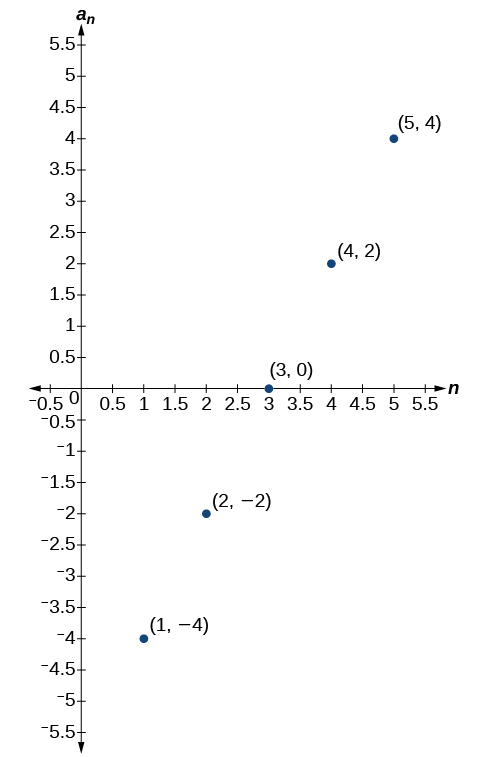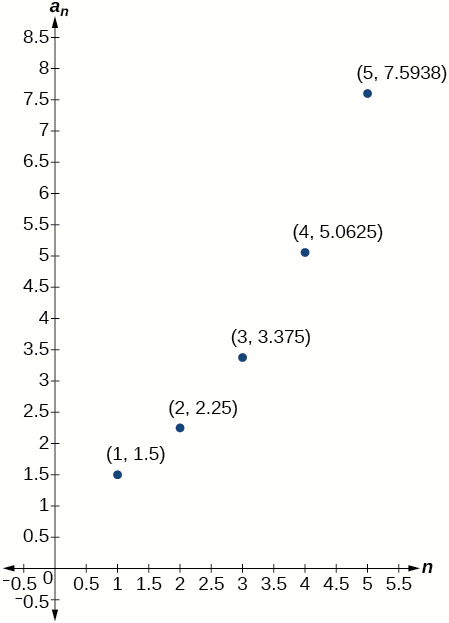The graph does not represent an arithmetic sequence.

For the following exercises, use the information provided to graph the first 5 terms of the arithmetic sequence.

${a}_{1}=0,d=4$

${a}_{1}=9;{a}_{n}={a}_{n-1}-10$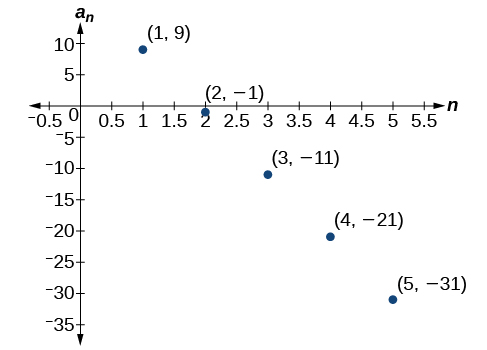${a}_{n}=-12+5n$

## Technology

For the following exercises, follow the steps to work with the arithmetic sequence ${a}_{n}=3n-2$ using a graphing calculator:

• Press [MODE]
• Select SEQ in the fourth line
• Select DOT in the fifth line
• Press [ENTER]
• Press [Y=]
• $n\text{Min}\text{\hspace{0.17em}}$ is the first counting number for the sequence. Set $\text{\hspace{0.17em}}n\text{Min}=1$
• $u\left(n\right)\text{\hspace{0.17em}}$ is the pattern for the sequence. Set $\text{\hspace{0.17em}}u\left(n\right)=3n-2$
• $u\left(n\text{Min)}\text{\hspace{0.17em}}$ is the first number in the sequence. Set $\text{\hspace{0.17em}}u\left(n\text{Min)}=1$
• Press [2ND] then [WINDOW] to go to TBLSET
• Set $\text{\hspace{0.17em}}\text{TblStart}=1$
• Set $\text{\hspace{0.17em}}\Delta \text{Tbl}=1$
• Set Indpnt: Auto and Depend: Auto
• Press [2ND] then [GRAPH] to go to the TABLE

What are the first seven terms shown in the column with the heading $u\left(n\right)\text{?}$

$1,4,7,10,13,16,19$

Use the scroll-down arrow to scroll to $n=50.$ What value is given for $u\left(n\right)\text{?}$

Press [WINDOW] . Set $\text{\hspace{0.17em}}n\text{Min}=1,n\text{Max}=5,x\text{Min}=0,x\text{Max}=6,y\text{Min}=-1,\text{\hspace{0.17em}}$ and $\text{\hspace{0.17em}}y\text{Max}=14.\text{\hspace{0.17em}}$ Then press [GRAPH] . Graph the sequence as it appears on the graphing calculator.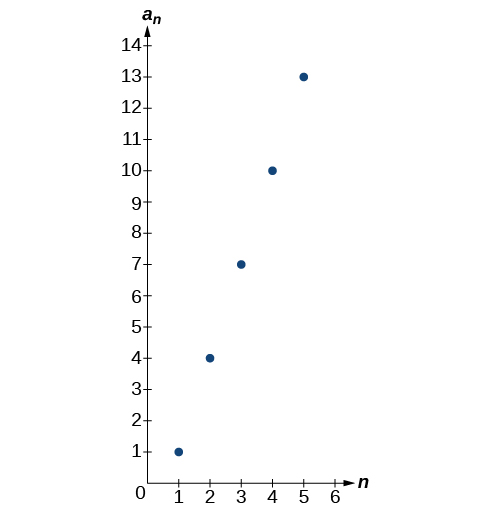For the following exercises, follow the steps given above to work with the arithmetic sequence ${a}_{n}=\frac{1}{2}n+5$ using a graphing calculator.

What are the first seven terms shown in the column with the heading $\text{\hspace{0.17em}}u\left(n\right)\text{\hspace{0.17em}}$ in the TABLE feature?

Graph the sequence as it appears on the graphing calculator. Be sure to adjust the WINDOW settings as needed.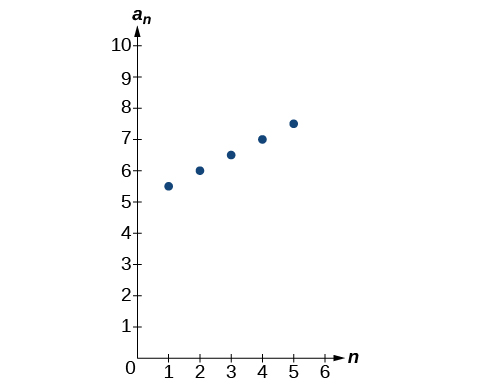## Extensions

Give two examples of arithmetic sequences whose 4 th terms are $9.$

Give two examples of arithmetic sequences whose 10 th terms are $206.$

Answers will vary. Examples: ${a}_{n}=20.6n$ and ${a}_{n}=2+20.4\mathrm{n.}$

Find the 5 th term of the arithmetic sequence $\left\{9b,5b,b,\dots \right\}.$

Find the 11 th term of the arithmetic sequence $\left\{3a-2b,a+2b,-a+6b\dots \right\}.$

${a}_{11}=-17a+38b$

At which term does the sequence $\left\{5.4,14.5,23.6,...\right\}$ exceed 151?

At which term does the sequence $\left\{\frac{17}{3},\frac{31}{6},\frac{14}{3},...\right\}$ begin to have negative values?

The sequence begins to have negative values at the 13 th term, ${a}_{13}=-\frac{1}{3}$

For which terms does the finite arithmetic sequence $\left\{\frac{5}{2},\frac{19}{8},\frac{9}{4},...,\frac{1}{8}\right\}$ have integer values?

Write an arithmetic sequence using a recursive formula. Show the first 4 terms, and then find the 31 st term.

Answers will vary. Check to see that the sequence is arithmetic. Example: Recursive formula: ${a}_{1}=3,{a}_{n}={a}_{n-1}-3.$ First 4 terms: $\begin{array}{ll}3,0,-3,-6\hfill & {a}_{31}=-87\hfill \end{array}$

Write an arithmetic sequence using an explicit formula. Show the first 4 terms, and then find the 28 th term.

#### Questions & Answers

write down the polynomial function with root 1/3,2,-3 with solution
if A and B are subspaces of V prove that (A+B)/B=A/(A-B)
write down the value of each of the following in surd form a)cos(-65°) b)sin(-180°)c)tan(225°)d)tan(135°)
Prove that (sinA/1-cosA - 1-cosA/sinA) (cosA/1-sinA - 1-sinA/cosA) = 4
what is the answer to dividing negative index
In a triangle ABC prove that. (b+c)cosA+(c+a)cosB+(a+b)cisC=a+b+c.
give me the waec 2019 questions
the polar co-ordinate of the point (-1, -1)
prove the identites sin x ( 1+ tan x )+ cos x ( 1+ cot x )= sec x + cosec x
tanh`(x-iy) =A+iB, find A and B
B=Ai-itan(hx-hiy)
Rukmini
what is the addition of 101011 with 101010
If those numbers are binary, it's 1010101. If they are base 10, it's 202021.
Jack
extra power 4 minus 5 x cube + 7 x square minus 5 x + 1 equal to zero
the gradient function of a curve is 2x+4 and the curve passes through point (1,4) find the equation of the curve
1+cos²A/cos²A=2cosec²A-1
test for convergence the series 1+x/2+2!/9x3ByByBy Nick SwainByByByBy